/* styles */
 table div table+table+table+table div table{width:100%;padding:0}table div table+table+table+table div table img{width:96.23%;padding:0;float:none}table div table+table+table+table div table td{width:100%;padding:0 1.88% 18px}/* styles */# Upcoming Important Dates

Tuesday, May 18 - LSC Meeting (link below)
Tuesday, May 25 - Friends of Northside Virtual Meeting (link below)

## News from the Counseling Office

▪ AP Exam Info: Info About Using Personal Computers, AP Checklist, Digital Practice Info, Exam Dates, AP Fees to be Paid for by CPS - Refunds to be Issued to Parents/Guardians
▪ Class of 2021 Spring Checklist
▪ Apprenticeships
▪ Summer Enrichment/Pre-College Programs
 ▪ AP Exam Info: Info About Using Personal Computers, AP Checklist, Digital Practice Info, Exam Dates, AP Fees to be Paid for by CPS - Refunds to be Issued to Parents/Guardians
 ▪ Class of 2021 Spring Checklist
 ▪ Apprenticeships
 ▪ Summer Enrichment/Pre-College Programs

## Annual Benefit Info, Graduation Events, Mental Health Help, Sports, & More

▪ Check out all of the Together but Apart Auction Listings: Our Silent Auction Closes Tonight at 9:00 pm
▪ Commencement & End of Year Information for Seniors - Graduation Fee, Senior Banquet, Calendar of Events
▪ Mental Health Resources
▪ Donations Needed for Student Resource Closet (Kato's Corner) - Items Can be Shipped
▪ Help Needed for Next School Year: FON Board, Website Assistance, and Email Communications
▪ NCP Weekly Updates via SchoolCNXT and via Email
▪ NCP Sports Schedule for This Week
▪ NCP Car Magnets and Window/Yard Signs Available
▪ Order Your 2020-2021 NCP Yearbook
▪ Ways for Parents to Stay in Touch/Volunteer/Support NCP/Social Media Links
 ▪ Check out all of the Together but Apart Auction Listings: Our Silent Auction Closes Tonight at 9:00 pm
 ▪ Commencement & End of Year Information for Seniors - Graduation Fee, Senior Banquet, Calendar of Events
 ▪ Mental Health Resources
 ▪ Donations Needed for Student Resource Closet (Kato's Corner) - Items Can be Shipped
 ▪ Help Needed for Next School Year: FON Board, Website Assistance, and Email Communications
 ▪ NCP Weekly Updates via SchoolCNXT and via Email
 ▪ NCP Sports Schedule for This Week
 ▪ NCP Car Magnets and Window/Yard Signs Available
 ▪ Order Your 2020-2021 NCP Yearbook
 ▪ Ways for Parents to Stay in Touch/Volunteer/Support NCP/Social Media Links
 table div table+table+table+table+table+table+table div table{width:100%;padding:0}table div table+table+table+table+table+table+table div table img{width:96.23%;padding:0;float:none}table div table+table+table+table+table+table+table div table td{width:100%;padding:0 1.88% 18px}/* styles */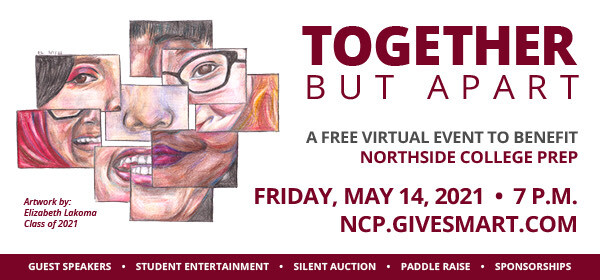# Thank You for Supporting Our Virtual Spring Benefit

Thank you so much for supporting "Together But Apart" virtual spring benefit on Friday, May 14! We appreciate your help raising funds for school programs not covered in the traditional CPS budget.

# The Benefit Video is Available for Viewing

We had a large number of parents who registered and watched the event. If you did not have a chance to register and/or see the video, we placed it on YouTube to view at your leisure. Please consider a donation as you are viewing it.

# Our Silent Auction Closes Tonight at 9:00 PM

Our silent auction will close TODAY, Sunday, May 16 at 9:00 pm. Find items available for purchase, with all proceeds benefiting the school. There is something for everyone in the family! (Think: a vacation, local gift cards and so much more!)

# Donations Towards Fund-a-Need, the General Fund, and Turf Field Will Remain Open Until May 23

Teacher Fund-A-Need, donations towards the turf field, and donations to the general fund will remain open until Sunday, May 23 at 9:00 pm.

For Fund-a-Need, consider donating in-whole or in-part to any item listed as needed by the school. Some items are small donation amounts and may be funded very quickly. Some items are larger donation amounts and can be funded by one generous family or multiple families.

 table div table+table+table+table+table+table+table+table+table+table+table+table+table+table+table div table{width:100%;padding:0}table div table+table+table+table+table+table+table+table+table+table+table+table+table+table+table div table img{width:96.23%;padding:0;float:none}table div table+table+table+table+table+table+table+table+table+table+table+table+table+table+table div table td{width:100%;padding:0 1.88% 18px}/* styles */# Commencement & End of Year Information for Seniors

## COMMENCEMENT CEREMONY - GRADUATION FEE INFO

Friday, June 18, 3:00 pm: Graduation will be at Soldier Field. Students will receive four tickets, and families will be assigned to zones for entry and exit. The graduation fee is \$75.00 and can be paid online. The fee must be paid by Friday, June 4th. For questions regarding fees, please contact our Business Manager, Ms. Espinoza at arespinoza@cps.edu.

## SENIOR BANQUET

Tuesday, June 15, time TBD: The Senior Banquet is an optional event and is an opportunity for our seniors to come together to enjoy a meal, an awards reception, and most importantly, to spend time with their classmates and teachers in person! The banquet will be held at Navy Pier during the day and students will have the option to meet at Navy Pier or ride a school bus from Northside.

Students should RSVP to attend the banquet by Friday, May 21 using the form sent by Ms. O’Hare. The cost is \$50.00 and can be paid online once their RSVP has been processed.

## SENIOR EVENTS

● Monday, June 14: Senior Picnic
● Tuesday, June 15: Senior Banquet at Navy Pier
● Wednesday, June 16: IDay (Virtual)
● Thursday, June 17: Graduation Rehearsal

## YARD SIGNS

Senior Yard Signs are available for pick up! Seniors may pick up their yard signs outside of the main office next week, May 10 - May 14.
● Hybrid Shift A: After school Monday & Tuesday
● Hybrid Shift B: After school Thursday & Friday
● Remote Learners: Wednesday from 8:00 am - 4:30 pm.

 table div table+table+table+table+table+table+table+table+table+table+table+table+table+table+table+table+table div table{width:100%;padding:0}table div table+table+table+table+table+table+table+table+table+table+table+table+table+table+table+table+table div table img{width:96.23%;padding:0;float:none}table div table+table+table+table+table+table+table+table+table+table+table+table+table+table+table+table+table div table td{width:100%;padding:0 1.88% 18px}/* styles */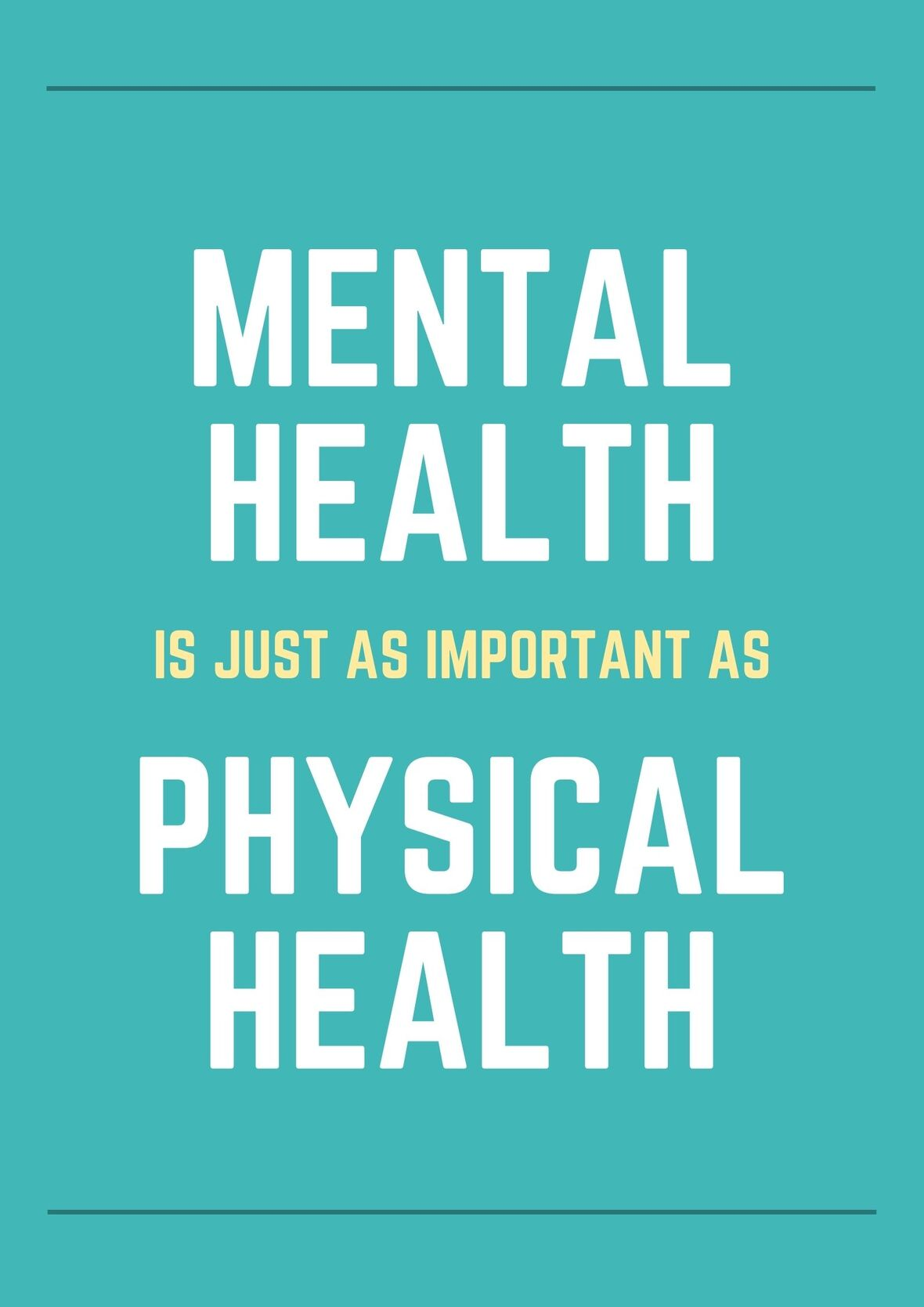# Mental Health Resources

With finals and AP Exams right around the corner, it is natural for our students to experience testing anxiety. Some things you can do to help your student cope if they are experiencing anxiety are help them plan their time appropriately, so they do not find themselves cramming the night before for anyone exam, encourage them to get plenty of rest in the upcoming weeks, and to make sure they are eating well and getting some exercise.

For more tips and help surrounding testing anxiety, please check out this resource.

 table div table+table+table+table+table+table+table+table+table+table+table+table+table+table+table+table+table+table+table div table{width:100%;padding:0}table div table+table+table+table+table+table+table+table+table+table+table+table+table+table+table+table+table+table+table div table img{width:96.23%;padding:0;float:none}table div table+table+table+table+table+table+table+table+table+table+table+table+table+table+table+table+table+table+table div table td{width:100%;padding:0 1.88% 18px}/* styles */# Using Personal Computers vs. School-Issued Chromebooks, AP Checklist, Digital Practice Info, Exam Dates, AP Fees to be Paid for by CPS - Refunds to be Issued to Parents/Guardians

AP exam season is swiftly approaching and here is some preliminary information. Please read this in its entirety so that you are fully aware of what is going on with AP Exams this year.

## Using a Personal Computer vs. CPS-Issued Chromebooks

Many students are requesting to use their personal computers. Because CPS has pushed out the test-taking app to school-issued Chromebook, it seems appropriate and easier to use. We are strongly encouraging your student to use the school-issued Chromebook.

Mr. Edin Ramovic is available at ERamovic1@cps.edu in case your child needs to exchange or swap old school-issued Chromebook. If that is the case and your child's Chromebook is busted, won't turn on, etc., then your student needs to reach out to Mr. Edin Ramovic immediately to set up an exchange! DO NOT DELAY!!!!

Using a Personal Computer
If your child chooses to use their personal computer, they can only use a desktop or laptop. Chromebooks not issued by CPS are not allowed. Students will need to download the app for testing onto their personal computers and follow those instructions. This should be done as soon as possible so that they can troubleshoot any issues. It is crucial that your student is logging in to the AP exam application and going through the setup process which includes logging in and setting up.

Here are the necessary steps to ensure your student is set up correctly if using their own personal computer. If they are not able to access any of the testing materials or able to follow procedures given to them by the College Board, then they certainly need to use the school-issued Chromebook.

## What to Do in Advance of the AP Exam Date

Because the majority of students will be taking their AP Exams digitally at home, there are a few things to know/do/remember. First thing first and most importantly, please be sure that the College Board account and email address for MyAP are correct/consistent. Some are using their personal email and others are using CPS's email. Just make sure it is consistent because the College Board will be sending them direct information regarding their AP exams. Please CONFIRM YOUR EMAIL ADDRESS <----Click here and review

If using the school-issued Chromebook, students should not log into their computer. From the start-up position, they will see three tabs on the lower-left corner of the screen. (Shut down) (Apps) (Add Person) - Select the Apps tab and click on 2021 Digital AP Exams. From there, run through the SETUP process and follow the instructions on the screen.

Four Steps to Take Digital AP Exams <---- Click here and review. They do not need to complete the first step because the APP is already installed onto their school-issued Chromebook.

2021 AP Exam Day Checklist <---REVIEW this checklist as MANY TIMES as you need to so that you are prepared to take your exam(s).

Getting Ready for Digital Exams <---Short PowerPoint

Exam Dates *AP exams in Illinois will be taken CDT (Central Daylight Time) During Administration 2, some exams begin at 11:00 am and others at 3:00 pm. Your child needs to look at the exam schedule to make sure they know what time the exam starts and how far in advance they should be ready to test!!!

Over the next two weeks, they should log into their MyAP account and run practice exams to ensure that they are aware of the necessary steps needed in order for them to be prepared to take their AP Exams. These are practice exams and they can enter and reset them as many times as needed.

I, along with their teachers, will be able to see if they have attempted to run a practice or not. Their teachers may also want to run a practice exam as well during class. THEY SHOULD NOT WAIT UNTIL DAYS BEFORE THEIR EXAM TO RUN THE PRACTICE EXAMS AND SET UP!!!! Their teachers, the College Board, or I will not be able to assist you because they postponed running the setup functions and practicing ahead of time! Remember, they can see if your student has done it or not!

I will be sending out separate emails to AP STEM test takers and AP WORLD LANGUAGE test takers!

Exam Fees Refunded
Finally, students/families will begin receiving a refund for paid AP exams. CPS (district) has opted to pay for exams again this year. I DO NOT know when you will receive it as there is only one person issuing the refunds for over 900 students. NO the exams are not canceled, just paid for! Be patient!

--
Alexander Hughes, M.S.
Professional School and College Counselor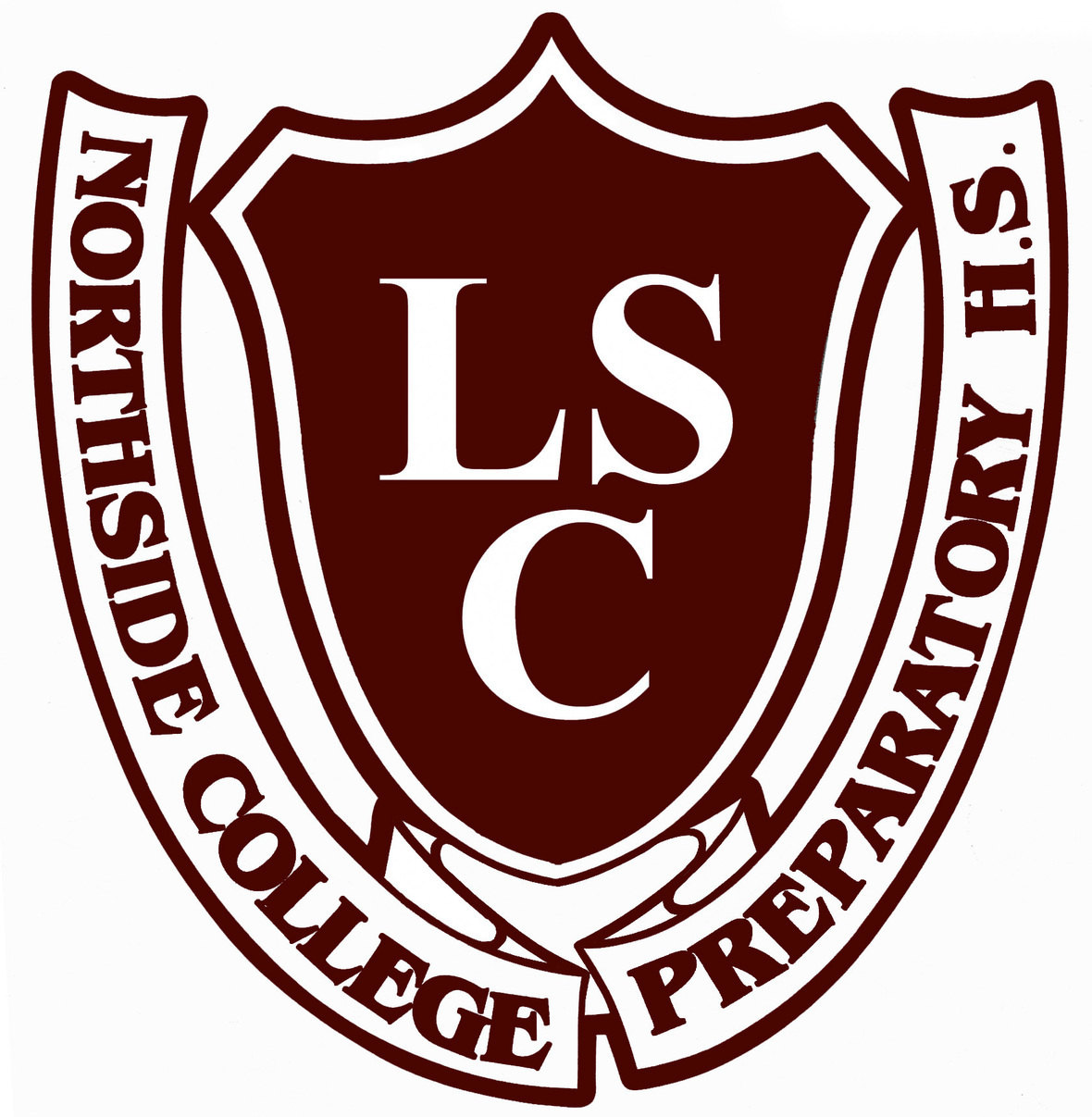# LSC Meeting Notice and Agenda

Tuesday, May 18
5:30 pm

Join Zoom Meeting
Meeting ID: 882 2176 0150
Passcode: 297331
One tap mobile
+13126266799,,88221760150#,,,,*297331# US (Chicago)

The agenda can be accessed here.

 table div table+table+table+table+table+table+table+table+table+table+table+table+table+table+table+table+table+table+table+table+table+table+table div table{width:100%;padding:0}table div table+table+table+table+table+table+table+table+table+table+table+table+table+table+table+table+table+table+table+table+table+table+table div table img{width:96.23%;padding:0;float:none}table div table+table+table+table+table+table+table+table+table+table+table+table+table+table+table+table+table+table+table+table+table+table+table div table td{width:100%;padding:0 1.88% 18px}/* styles */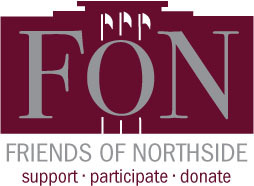https://meet.google.com/gte-adsy-jqh

# Friends of Northside

Tuesday, May 25

Please join Friends of Northside for their upcoming meeting.

Friends of Northside (FON) is the “one-stop shop” for NCP families to get involved. FON facilitates communication between parents and the school, organizes parent volunteers, plans and sponsors social/educational events, and raises much-needed funds to bridge the gap due to CPS budget cuts. FON supports the school’s mission to maintain and enhance the learning experience for all students of Northside College Prep High School.

To find out more about Friends of Northside, please visit their website HERE.

 table div table+table+table+table+table+table+table+table+table+table+table+table+table+table+table+table+table+table+table+table+table+table+table+table+table div table{width:100%;padding:0}table div table+table+table+table+table+table+table+table+table+table+table+table+table+table+table+table+table+table+table+table+table+table+table+table+table div table img{width:96.23%;padding:0;float:none}table div table+table+table+table+table+table+table+table+table+table+table+table+table+table+table+table+table+table+table+table+table+table+table+table+table div table td{width:100%;padding:0 1.88% 18px}/* styles */# Donations Needed for Student Resource Closet (Kato's Corner)

Friends of Northside has a resource closet available to NCP students. We are in need of:

▪ \$10 gift cards to local restaurants for kids who have to stay late and don't have money for supper (McDonald's, Subway, Jimmy John's, etc.)
▪ Hair Products for a variety of hair/gender
▪ Shampoo
▪ Conditioner
▪ Dandruff shampoo/conditioner
▪ 2-in-1 shampoo/conditioner
▪ Toothbrushes
▪ Women's specific products
▪ Shave gel
▪ Deodorant
▪ Underwear
▪ Socks
▪ Body wash
▪ Lotion
▪ Non-perishable food items individually wrapped (i.e. granola bars)
▪ Pants / shorts (gender-neutral)
▪ School supplies
▪ Highlighters
▪ Compasses
▪ Scissors
▪ Colored pencils
▪ Rain gear (umbrellas/ponchos/raincoats)
▪ Calculators
▪ Index cards
▪ Notebooks
▪ Reusable water bottles
 ▪ \$10 gift cards to local restaurants for kids who have to stay late and don't have money for supper (McDonald's, Subway, Jimmy John's, etc.)
 ▪ Hair Products for a variety of hair/gender
 ▪ Shampoo
 ▪ Conditioner
 ▪ Dandruff shampoo/conditioner
 ▪ 2-in-1 shampoo/conditioner
 ▪ Toothbrushes
 ▪ Women's specific products
 ▪ Shave gel
 ▪ Deodorant
 ▪ Underwear
 ▪ Socks
 ▪ Body wash
 ▪ Lotion
 ▪ Non-perishable food items individually wrapped (i.e. granola bars)
 ▪ Pants / shorts (gender-neutral)
 ▪ School supplies
 ▪ Highlighters
 ▪ Compasses
 ▪ Scissors
 ▪ Colored pencils
 ▪ Rain gear (umbrellas/ponchos/raincoats)
 ▪ Calculators
 ▪ Index cards
 ▪ Notebooks
 ▪ Reusable water bottles

All items can be shipped directly to Ms. Mathews at NCP, 5501 N. Kedzie Ave., Chicago IL 60625.

Contact Kristen Werries Collier with any questions.

 table div table+table+table+table+table+table+table+table+table+table+table+table+table+table+table+table+table+table+table+table+table+table+table+table+table+table+table div table{width:100%;padding:0}table div table+table+table+table+table+table+table+table+table+table+table+table+table+table+table+table+table+table+table+table+table+table+table+table+table+table+table div table img{width:96.23%;padding:0;float:none}table div table+table+table+table+table+table+table+table+table+table+table+table+table+table+table+table+table+table+table+table+table+table+table+table+table+table+table div table td{width:100%;padding:0 1.88% 18px}/* styles */# Help Needed: FON Board, Website Assistance, and Email Communications

FON is seeking volunteers to:
* Join the Friends of Northside Board;
* Assist in writing the "This Week at Northside" Sunday E-Blast
* and many more positions.

No experience required.

If you're interested in joining the board or helping with any of the positions listed, please email Matt Schechter, president of FON, at matthew.d.schechter@gmail.com.

# Become a FON Board Member

Friends of Northside (FON) is a parent organization that works to support the Northside community through fundraising and volunteerism. All parents and guardians are automatically members of FON. Board positions are available for those who would like to take a more active role.

FON's fundraising seeks to raise money with the goals of replacing lost funding from CPS, supporting the school’s mission, and maintaining and enhancing the learning experience for all NCP students. FON efforts include the Annual Appeal, Spring Benefit, and Cubby Walk Walk-a-thon, among others.

FON also provides volunteers and hospitality to the NCP community. It plans social/educational programs, recruits volunteers for various NCP events such as the Open House, distributes this weekly email communication, and maintains the student directory.

To see all the volunteer opportunities listed for 2020-2021 please click HERE to view them on the Friends of Northside website.

 table div table+table+table+table+table+table+table+table+table+table+table+table+table+table+table+table+table+table+table+table+table+table+table+table+table+table+table+table+table div table{width:100%;padding:0}table div table+table+table+table+table+table+table+table+table+table+table+table+table+table+table+table+table+table+table+table+table+table+table+table+table+table+table+table+table div table img{width:96.23%;padding:0;float:none}table div table+table+table+table+table+table+table+table+table+table+table+table+table+table+table+table+table+table+table+table+table+table+table+table+table+table+table+table+table div table td{width:100%;padding:0 1.88% 18px}/* styles */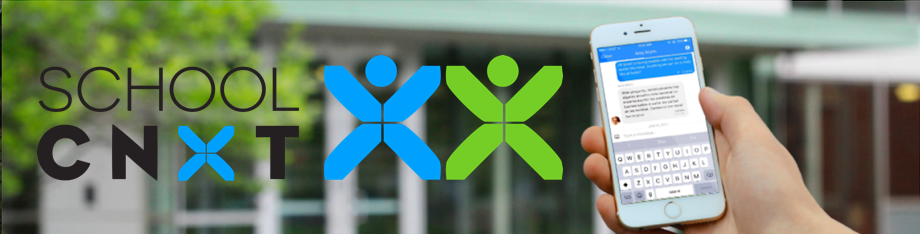# NCP Weekly Updates to Parents

NCP now sends a consolidated update to parents every Friday with important information. Parents should be on the lookout for these updates, which will be posted via School CNXT and emailed to families.

You can gain access by using this tool on Northside's website.

If you are having problems with SchoolCNXT, please contact the main office from 7:30 am - 3:15 pm at 773-534-3954, or you can email the NCP school clerk, Mary Grasz, at mtgrasz@cpu.edu.

 table div table+table+table+table+table+table+table+table+table+table+table+table+table+table+table+table+table+table+table+table+table+table+table+table+table+table+table+table+table+table+table+table div table{width:100%;padding:0}table div table+table+table+table+table+table+table+table+table+table+table+table+table+table+table+table+table+table+table+table+table+table+table+table+table+table+table+table+table+table+table+table div table img{width:96.23%;padding:0;float:none}table div table+table+table+table+table+table+table+table+table+table+table+table+table+table+table+table+table+table+table+table+table+table+table+table+table+table+table+table+table+table+table+table div table td{width:100%;padding:0 1.88% 18px}/* styles */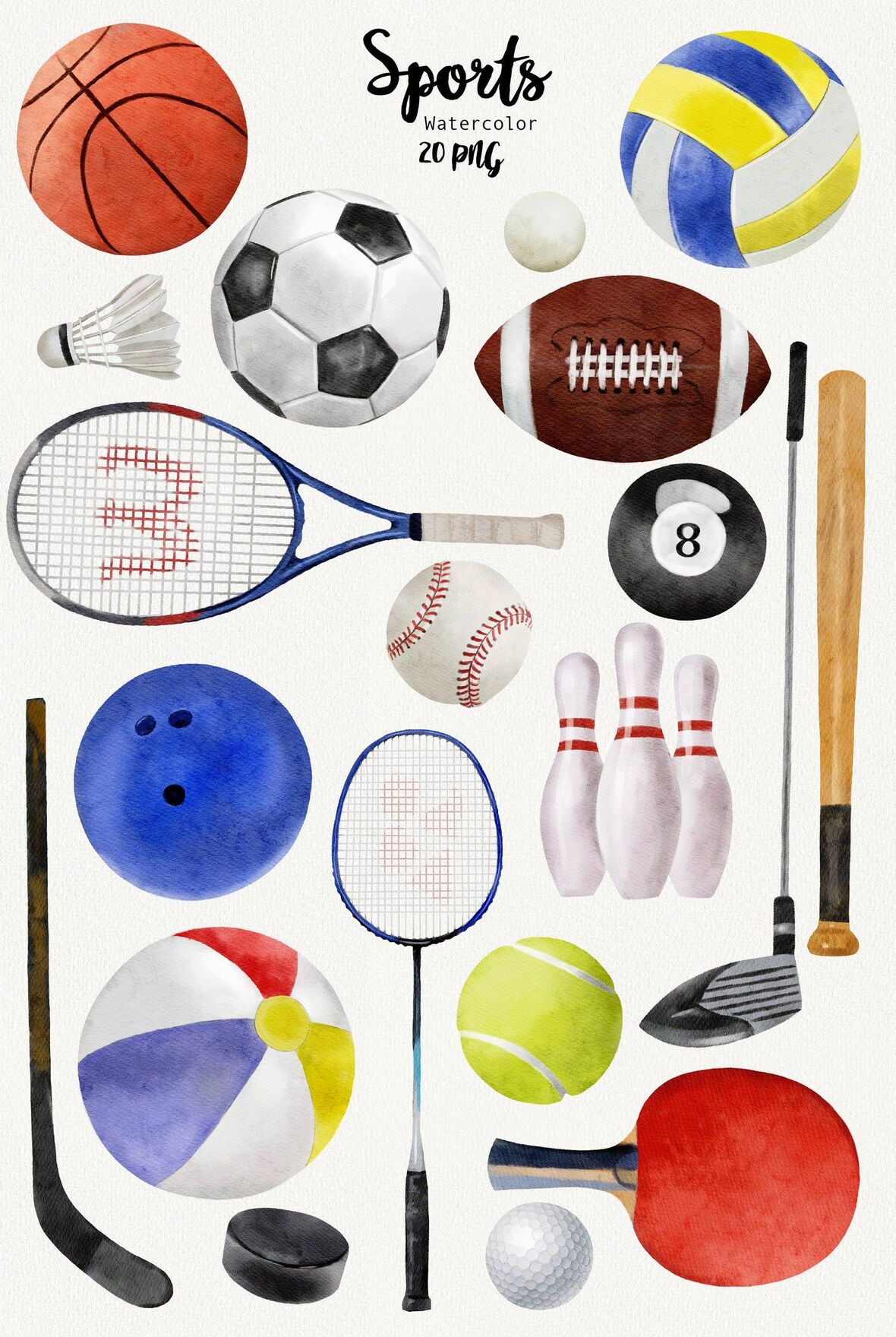# NCP Sports Schedule for This Week

Boys Volleyball
▪ Monday, May 17 vs. Lakeview (away) at 5:00 pm
▪ Wednesday, May 19 vs. North Grand at NCP at 5:00 pm
▪ Thursday, May 20 vs. Latin at NCP at 6:00 pm

Girls Soccer
▪ Wednesday, May 19 vs. Taft (away) JV at 5:00 pm

Boys Baseball
▪ Monday, May 17 vs. Lincoln Park (Home/River Park) at 4:30 pm

Girls Softball
▪ Monday, May 17 vs. Jones (away) at 4:30 pm

Boys Lacrosse
▪ Monday, May 17 vs. Lincoln Park at NCP (V) at 5:00 pm

Girls Lacrosse
▪ Tuesday, May 18 vs. Jones (home) JV at 5:00 pm
▪ Thursday, May 20 vs. Trinity (home) V at 5:00 pm, JV at 6:15 pm

 table div table+table+table+table+table+table+table+table+table+table+table+table+table+table+table+table+table+table+table+table+table+table+table+table+table+table+table+table+table+table+table+table+table+table div table{width:100%;padding:0}table div table+table+table+table+table+table+table+table+table+table+table+table+table+table+table+table+table+table+table+table+table+table+table+table+table+table+table+table+table+table+table+table+table+table div table img{width:96.23%;padding:0;float:none}table div table+table+table+table+table+table+table+table+table+table+table+table+table+table+table+table+table+table+table+table+table+table+table+table+table+table+table+table+table+table+table+table+table+table div table td{width:100%;padding:0 1.88% 18px}/* styles */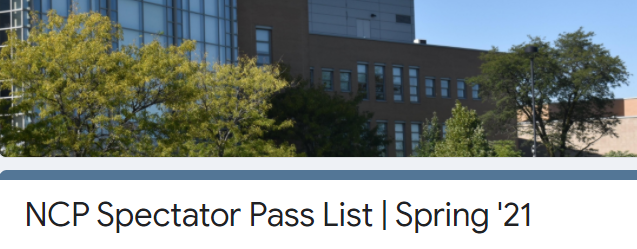# Update on Outdoor Attendance

At this time, CPS is not allowing spectators for indoor sports, however, limited spectators will be allowed for outdoor activities.

Per CPS guidelines: For games held outdoors, student-athletes and coaches may add the names of no more than two spectators to an approved pass list.

Spectators are restricted to family members only (i.e., parent/guardian, grandparent, sibling). Schools may ONLY allow entrance to individuals listed on the APPROVED pass list.

If you would like the opportunity to attend your NCP athlete's outdoor games, please identify the names of TWO family members for the NCP Spectator Pass List by completing this form.

Any spectators NOT on this list will NOT be allowed admission to NCP games.

In addition to this list, spectators will also need to:

1. Complete the required CPS Health Screener BY 2:00 pm on weekdays and 8:45 am on weekends.
2. Pass a temperature check.
3. Wear a CFC (cloth face covering or mask) at all times.
4. Maintain social distancing of 6 ft at all times with spectators from different households.
 1 Complete the required CPS Health Screener BY 2:00 pm on weekdays and 8:45 am on weekends.
 2 Pass a temperature check.
 3 Wear a CFC (cloth face covering or mask) at all times.
 4 Maintain social distancing of 6 ft at all times with spectators from different households.
 table div table+table+table+table+table+table+table+table+table+table+table+table+table+table+table+table+table+table+table+table+table+table+table+table+table+table+table+table+table+table+table+table+table+table+table+table+table div table{width:100%;padding:0}table div table+table+table+table+table+table+table+table+table+table+table+table+table+table+table+table+table+table+table+table+table+table+table+table+table+table+table+table+table+table+table+table+table+table+table+table+table div table img{width:96.23%;padding:0;float:none}table div table+table+table+table+table+table+table+table+table+table+table+table+table+table+table+table+table+table+table+table+table+table+table+table+table+table+table+table+table+table+table+table+table+table+table+table+table div table td{width:100%;padding:0 1.88% 18px}/* styles */# Class of 2021: Spring Checklist

Class of 2021 Parents,

The end of the school year is rapidly approaching. With all of the duties and responsibilities your student is expected to complete between now and June, the counseling department would like to encourage your family to discuss the following checklists and create a plan to address the relevant action items.

Consider completing the following action items on the Class of 2021: Check-out List (click here) as well as the additional action items listed on the Spring Checklist (click here) to assist you with remaining on track for the second semester.

If you would like to schedule a one on one with Ms. Moore via Calendly, visit https://calendly.com/mcmoore6/ or contact her via e-mail at mcmoore6@cps.edu. Appointments are available during lunch blocks, after school, and during parent-teacher conferences in April.

 table div table+table+table+table+table+table+table+table+table+table+table+table+table+table+table+table+table+table+table+table+table+table+table+table+table+table+table+table+table+table+table+table+table+table+table+table+table+table+table div table{width:100%;padding:0}table div table+table+table+table+table+table+table+table+table+table+table+table+table+table+table+table+table+table+table+table+table+table+table+table+table+table+table+table+table+table+table+table+table+table+table+table+table+table+table div table img{width:96.23%;padding:0;float:none}table div table+table+table+table+table+table+table+table+table+table+table+table+table+table+table+table+table+table+table+table+table+table+table+table+table+table+table+table+table+table+table+table+table+table+table+table+table+table+table div table td{width:100%;padding:0 1.88% 18px}/* styles */# Apprenticeships

The Apprenticeship Pathway involves getting paid as you learn a highly skilled profession. You’ll get an inside look at what life is really like in a certain industry or at a specific company and earn a living wage. Different industries offer apprenticeship programs, including:
▪ Construction
▪ Manufacturing
▪ Health care
▪ Information technology
▪ Transportation
▪ Energy
▪ Other

If an apprenticeship is a postsecondary option that interests you, consider taking the next steps:

## Is an apprenticeship the right plan for you?

▪ Meet with your counselor, teachers, family members, and other advisors to get help as you explore if an apprenticeship is right for you.
 ▪ Meet with your counselor, teachers, family members, and other advisors to get help as you explore if an apprenticeship is right for you.

## Explore apprenticeships

▪ Search for opportunities using Apprenticeship Finder or the Illinois Department of Employment Security (IDES) website. You can also search under Apprenticeship "related links” at cps.edu/LearnPlanSucceed.
 ▪ Search for opportunities using Apprenticeship Finder or the Illinois Department of Employment Security (IDES) website. You can also search under Apprenticeship "related links” at cps.edu/LearnPlanSucceed.

## Apply for Apprenticeship Opportunities

▪ The City Colleges of Chicago website offers apprenticeship programs that can take you from student to employee, opening the door into several of Chicago’s leading businesses.
 ▪ The City Colleges of Chicago website offers apprenticeship programs that can take you from student to employee, opening the door into several of Chicago’s leading businesses.
 table div table+table+table+table+table+table+table+table+table+table+table+table+table+table+table+table+table+table+table+table+table+table+table+table+table+table+table+table+table+table+table+table+table+table+table+table+table+table+table+table+table div table{width:100%;padding:0}table div table+table+table+table+table+table+table+table+table+table+table+table+table+table+table+table+table+table+table+table+table+table+table+table+table+table+table+table+table+table+table+table+table+table+table+table+table+table+table+table+table div table img{width:96.23%;padding:0;float:none}table div table+table+table+table+table+table+table+table+table+table+table+table+table+table+table+table+table+table+table+table+table+table+table+table+table+table+table+table+table+table+table+table+table+table+table+table+table+table+table+table+table div table td{width:100%;padding:0 1.88% 18px}/* styles */# Summer Enrichment/Pre-College Programs

In addition to the virtual information sessions Northside Prep is offering after school daily and during the school day on Wednesdays (click HERE), the counseling department would like to share additional information about information sessions for summer enrichment opportunities.

Are you and your student interested in learning about enrichment opportunities or pre-college programs to engage in during the spring or summer? Would you like to build your resume and enhance your academic, personal, or social skills? If the answer is yes, consider reviewing the following resources to assist your family with identifying the best fit enrichment opportunities for your student:

 table div table+table+table+table+table+table+table+table+table+table+table+table+table+table+table+table+table+table+table+table+table+table+table+table+table+table+table+table+table+table+table+table+table+table+table+table+table+table+table+table+table+table+table+table div table{width:100%;padding:0}table div table+table+table+table+table+table+table+table+table+table+table+table+table+table+table+table+table+table+table+table+table+table+table+table+table+table+table+table+table+table+table+table+table+table+table+table+table+table+table+table+table+table+table+table div table img{width:96.23%;padding:0;float:none}table div table+table+table+table+table+table+table+table+table+table+table+table+table+table+table+table+table+table+table+table+table+table+table+table+table+table+table+table+table+table+table+table+table+table+table+table+table+table+table+table+table+table+table+table div table td{width:100%;padding:0 1.88% 18px}/* styles */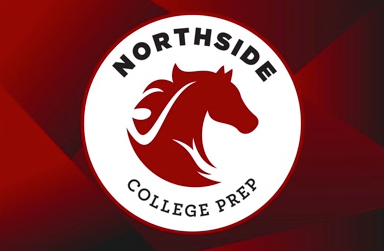# NCP Car Magnets and Window/Yard Signs Available

Friends of Northside is pleased to offer NCP car magnets for sale and to reopen orders for NCP yard/window signs. Show your school pride!

If you are interested, please click the button below. Each time we reach minimums for each item we will send for production and notify you of pickup timing. We anticipate two print runs for magnets and at least one for signs this school year. Go Mustangs!

Other swag is made available via the Saddle Shop directly via NCP; timing varies for their online pop-ups.

 table div table+table+table+table+table+table+table+table+table+table+table+table+table+table+table+table+table+table+table+table+table+table+table+table+table+table+table+table+table+table+table+table+table+table+table+table+table+table+table+table+table+table+table+table+table+table+table div table{width:100%;padding:0}table div table+table+table+table+table+table+table+table+table+table+table+table+table+table+table+table+table+table+table+table+table+table+table+table+table+table+table+table+table+table+table+table+table+table+table+table+table+table+table+table+table+table+table+table+table+table+table div table img{width:96.23%;padding:0;float:none}table div table+table+table+table+table+table+table+table+table+table+table+table+table+table+table+table+table+table+table+table+table+table+table+table+table+table+table+table+table+table+table+table+table+table+table+table+table+table+table+table+table+table+table+table+table+table+table div table td{width:100%;padding:0 1.88% 18px}/* styles */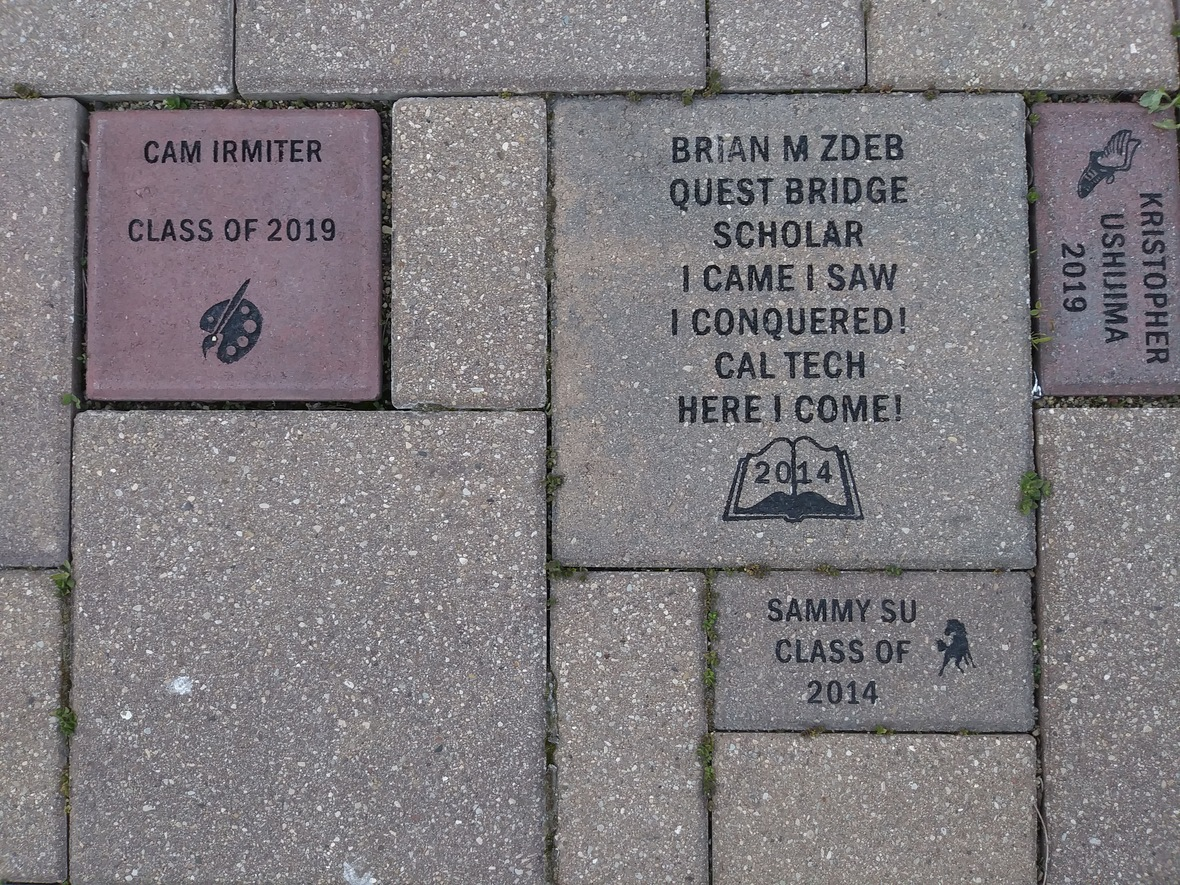Consider buying a custom NCP Brick for your child this year. Your brick, engraved with a personal message, will become a permanent part of the Northside College Prep Celestial Garden Walkway.

Your brick may be purchased in three sizes:

▪ 4" x 8" = \$50
▪ 8" x 8" = \$100
▪ 12" x 12" = \$150

Friends of Northside will provide a gift certificate acknowledging the purchase. Janet Jasmer will assist with the process and can answer any questions at jjasmer@atproperties.com.

 table div table+table+table+table+table+table+table+table+table+table+table+table+table+table+table+table+table+table+table+table+table+table+table+table+table+table+table+table+table+table+table+table+table+table+table+table+table+table+table+table+table+table+table+table+table+table+table+table+table div table{width:100%;padding:0}table div table+table+table+table+table+table+table+table+table+table+table+table+table+table+table+table+table+table+table+table+table+table+table+table+table+table+table+table+table+table+table+table+table+table+table+table+table+table+table+table+table+table+table+table+table+table+table+table+table div table img{width:96.23%;padding:0;float:none}table div table+table+table+table+table+table+table+table+table+table+table+table+table+table+table+table+table+table+table+table+table+table+table+table+table+table+table+table+table+table+table+table+table+table+table+table+table+table+table+table+table+table+table+table+table+table+table+table+table div table td{width:100%;padding:0 1.88% 18px}/* styles */table.module-49{width:68.87%;padding:0}table div table+table+table+table+table+table+table+table+table+table+table+table+table+table+table+table+table+table+table+table+table+table+table+table+table+table+table+table+table+table+table+table+table+table+table+table+table+table+table+table+table+table+table+table+table+table+table+table+table+table div table{width:68.87%;float:none;margin-left:auto;margin-right:auto;padding:0}table div table+table+table+table+table+table+table+table+table+table+table+table+table+table+table+table+table+table+table+table+table+table+table+table+table+table+table+table+table+table+table+table+table+table+table+table+table+table+table+table+table+table+table+table+table+table+table+table+table+table div table a{border:0 none;text-decoration:none}table div table+table+table+table+table+table+table+table+table+table+table+table+table+table+table+table+table+table+table+table+table+table+table+table+table+table+table+table+table+table+table+table+table+table+table+table+table+table+table+table+table+table+table+table+table+table+table+table+table+table div table img{width:100%!important;border:0 none;text-decoration:none}table div table+table+table+table+table+table+table+table+table+table+table+table+table+table+table+table+table+table+table+table+table+table+table+table+table+table+table+table+table+table+table+table+table+table+table+table+table+table+table+table+table+table+table+table+table+table+table+table+table+table div table td{width:100%;padding:0}/* styles */

# Order a Yearbook Today!

Interested in purchasing a copy of the 2020-2021 Stampede, NCP's yearbook? Click HERE to start your order! And if you didn't get a chance to purchase a copy of the 2019-2020 yearbook, you now have a change to buy one of those, too! Just email Ms. McCreedy at mkmccreedy@cps.edu after you've completed your 2020-2021 order.

Copies are now \$55.

 table div table+table+table+table+table+table+table+table+table+table+table+table+table+table+table+table+table+table+table+table+table+table+table+table+table+table+table+table+table+table+table+table+table+table+table+table+table+table+table+table+table+table+table+table+table+table+table+table+table+table+table+table+table div table{width:100%;padding:0}table div table+table+table+table+table+table+table+table+table+table+table+table+table+table+table+table+table+table+table+table+table+table+table+table+table+table+table+table+table+table+table+table+table+table+table+table+table+table+table+table+table+table+table+table+table+table+table+table+table+table+table+table+table div table img{width:96.23%;padding:0;float:none}table div table+table+table+table+table+table+table+table+table+table+table+table+table+table+table+table+table+table+table+table+table+table+table+table+table+table+table+table+table+table+table+table+table+table+table+table+table+table+table+table+table+table+table+table+table+table+table+table+table+table+table+table+table div table td{width:100%;padding:0 1.88% 18px}/* styles */# Support Northside - Shop AmazonSmile

AmazonSmile is a simple and automatic way to support Northside every time you shop.

Please note that our AmazonSmile name is Friends of "North" "Side" (North Side - two words at AmazonSmile).

 table div table+table+table+table+table+table+table+table+table+table+table+table+table+table+table+table+table+table+table+table+table+table+table+table+table+table+table+table+table+table+table+table+table+table+table+table+table+table+table+table+table+table+table+table+table+table+table+table+table+table+table+table+table+table+table div table{width:100%;padding:0}table div table+table+table+table+table+table+table+table+table+table+table+table+table+table+table+table+table+table+table+table+table+table+table+table+table+table+table+table+table+table+table+table+table+table+table+table+table+table+table+table+table+table+table+table+table+table+table+table+table+table+table+table+table+table+table div table img{width:96.23%;padding:0;float:none}table div table+table+table+table+table+table+table+table+table+table+table+table+table+table+table+table+table+table+table+table+table+table+table+table+table+table+table+table+table+table+table+table+table+table+table+table+table+table+table+table+table+table+table+table+table+table+table+table+table+table+table+table+table+table+table div table td{width:100%;padding:0 1.88% 18px}/* styles */# Follow Us on Social Media

 table div table+table+table+table+table+table+table+table+table+table+table+table+table+table+table+table+table+table+table+table+table+table+table+table+table+table+table+table+table+table+table+table+table+table+table+table+table+table+table+table+table+table+table+table+table+table+table+table+table+table+table+table+table+table+table+table+table div table{width:100%;padding:0}table div table+table+table+table+table+table+table+table+table+table+table+table+table+table+table+table+table+table+table+table+table+table+table+table+table+table+table+table+table+table+table+table+table+table+table+table+table+table+table+table+table+table+table+table+table+table+table+table+table+table+table+table+table+table+table+table+table div table img{width:96.23%;padding:0;float:none}table div table+table+table+table+table+table+table+table+table+table+table+table+table+table+table+table+table+table+table+table+table+table+table+table+table+table+table+table+table+table+table+table+table+table+table+table+table+table+table+table+table+table+table+table+table+table+table+table+table+table+table+table+table+table+table+table+table div table td{width:100%;padding:0 1.88% 18px}/* styles */table div table+table+table+table+table+table+table+table+table+table+table+table+table+table+table+table+table+table+table+table+table+table+table+table+table+table+table+table+table+table+table+table+table+table+table+table+table+table+table+table+table+table+table+table+table+table+table+table+table+table+table+table+table+table+table+table+table+table+table div table{width:100%;padding:0}table div table+table+table+table+table+table+table+table+table+table+table+table+table+table+table+table+table+table+table+table+table+table+table+table+table+table+table+table+table+table+table+table+table+table+table+table+table+table+table+table+table+table+table+table+table+table+table+table+table+table+table+table+table+table+table+table+table+table+table div table img{width:96.23%;padding:0;float:none}table div table+table+table+table+table+table+table+table+table+table+table+table+table+table+table+table+table+table+table+table+table+table+table+table+table+table+table+table+table+table+table+table+table+table+table+table+table+table+table+table+table+table+table+table+table+table+table+table+table+table+table+table+table+table+table+table+table+table+table div table td{width:100%;padding:0 1.88% 18px}/* styles */